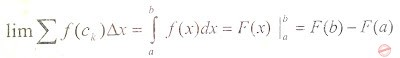# Lecture in Definite Integrals

(Last Updated On: March 2, 2019)Definite integrals are used to find the area between the graph of a function and the x-axis. If a continuous function f(x) is positive over the domain , then the area under its graph isWhere:

• F(x) is the integral of f(x);
• F(b) is the value of the integral at the upper limit, x=b; and
• F(a) is the value of the integral at the lower limit, x=a.

Note: It does not involve a constant of integration (arbitrary constant) and it gives us a definite value (a number) at the end of the calculation.

### Properties of the Definite Integral:

• Integral of a constant:

(a)

(b)• Linearity:

(a)(b)if c is a constant.

(a)(b)(c)If a < b then it is convenient to define

• Comparison:If 0 < f(x) < g(x) for all x in [a, b],

### The Evaluation Theorem

If is a continuous function and F is an antiderivative of f,   F'(x) = f(x), then

Example: Using the Theorem, Find the value of  .

• Applying what you learned, an antiderivative of x2 is
• Then, evaluate and substitute the limit.
• = with limit 0 to 1 =

At this point, you are ready to answer some problems involving definite integral. Follow the link to start. Definite Integral – Set 1 Problems

If you have some clarifications. Let me know.

credit: Renato E. Apa-ap, et al.

P inoyBIX educates thousands of reviewers and students a day in preparation for their board examinations. Also provides professionals with materials for their lectures and practice exams. Help me go forward with the same spirit.

“Will you subscribe today via YOUTUBE?”

Subscribe

• Full Content Access Exclusive to Premium members

## PINOYBIX FREEBIES FOR PREMIUM MEMBERSHIP:

• CIVIL ENGINEERING REVIEWER
• CIVIL SERVICE EXAM REVIEWER
• CRIMINOLOGY REVIEWER
• ELECTRONICS ENGINEERING REVIEWER (ECE/ECT)
• ELECTRICAL ENGINEERING & RME REVIEWER
• FIRE OFFICER EXAMINATION REVIEWER
• LET REVIEWER
• MASTER PLUMBER REVIEWER
• MECHANICAL ENGINEERING REVIEWER
• NAPOLCOM REVIEWER

## FOR A LIMITED TIME

If you subscribe for PREMIUM today!